### TRUE Function Examples – Excel & Google Sheets

This Tutorial demonstrates how to use the Excel TRUE Function in Excel to return the logical value TRUE.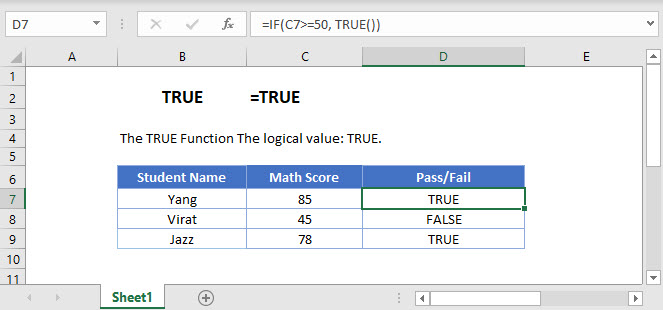## TRUE Function Overview

The TRUE Function The logical value: TRUE.

To use the TRUE Excel Worksheet Function, select a cell and type: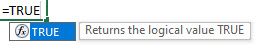(Notice how the formula inputs appear)

## TRUE Function Syntax and Inputs:

AutoMacro - VBA Code Generator

## TRUE Function

The TRUE Function returns a logical value of TRUE.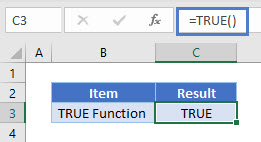## TRUE Function & IF Function

Generally the only time this function is used is within an IF Function to output the BOOLEAN value TRUE.

The boolean value TRUE is different from a text string “TRUE”.

First, Excel treats TRUE = 1 and FALSE = 0 .

By multiplying these boolean values with a result, you can output the TRUE if condition is true or FALSE if otherwise. Read more about the FALSE Function here.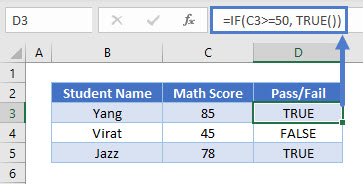Also, these boolean values can be fed directly into an IF Statement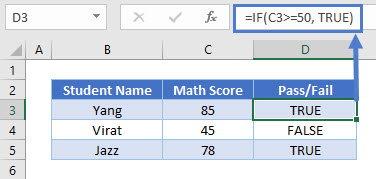## TRUE Function & NOT Function

The TRUE Function can also be used with the NOT Function.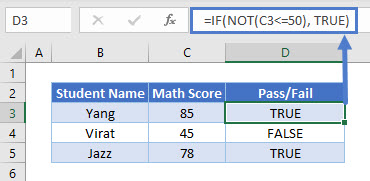## TRUE in Google Sheets

The TRUE Function works exactly the same in Google Sheets as in Excel: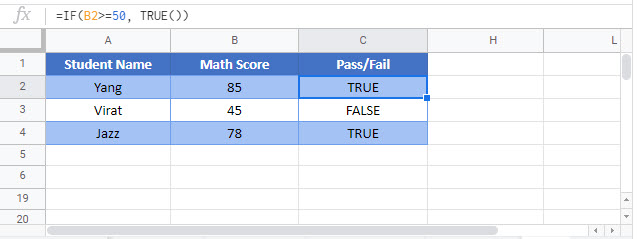## Additional Notes

To use the logical values TRUE or FALSE you can simply type “true” or “false”.

Return to the List of all Functions in Excel

## Excel Practice Worksheet

Practice Excel functions and formulas with our 100% free practice worksheets!

• Automatically Graded Exercises
• Learn Excel, Inside Excel!